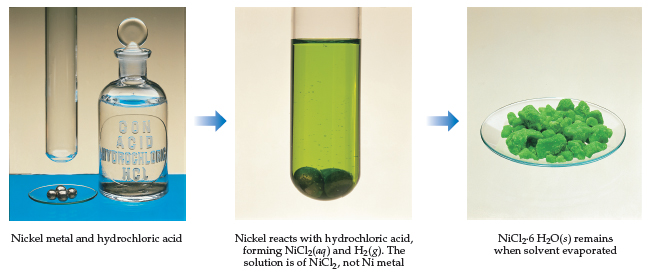# Problem: The reaction between nickel metal and hydrochloric acid is not a simple dissolution. The product is NiCl2 6H2O(s), nickel(II) chloride hexahydrate, which has exactly 6 waters of hydration in the crystal lattice for every nickel ion.What is the molar mass of nickel chloride hexahydrate, NiCl2 6H2O(s)?

###### FREE Expert Solution

We’re asked to determine the molar mass of nickel chloride hexahydrate, NiCl2•6H2O(s).

Recall that the molar mass of a compound is the sum of the atomic mass of each element in the compound.

**Multiply each atomic mass by its subscript

The subscript indicates the number of each element in the formula:

• When an element has no subscript = 1
98% (203 ratings)###### Problem DetailsThe reaction between nickel metal and hydrochloric acid is not a simple dissolution. The product is NiCl2 6H2O(s), nickel(II) chloride hexahydrate, which has exactly 6 waters of hydration in the crystal lattice for every nickel ion.

What is the molar mass of nickel chloride hexahydrate, NiCl2 6H2O(s)?